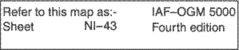# series

(redirected from granulocytic series)
Also found in: Dictionary, Thesaurus, Medical, Financial.

## series,

in mathematics, indicated sum of a sequencesequence,
in mathematics, ordered set of mathematical quantities called terms. A sequence is said to be known if a formula can be given for any particular term using the preceding terms or using its position in the sequence.
of terms. A series may be finite or infinite. A finite series contains a definite number of terms whose sum can be found by various methods. An infinite series is a sum of infinitely many terms, e.g., the infinite series 1-2 + 1-4 + 1-8 + 1-16 + … . The dots mean that the remaining terms are formed according to the rule made evident by the first few terms, in this case doubling the denominator of the preceding term to form that of the next term; the nth term of this series is ( 1-2)n. Some infinite series converge to a certain value called its limit; i.e., as one adds together progressively more terms, these sums (called the partial sums of the series) form a sequence of values that progressively approach the limit. For example, the series given above converges to the value 1 because the partial sums form the sequence 1-2, 3-4, 7-8, 15-16, … . Many series, however, do not converge, i.e., have no value that their partial sums approach. Such a series is 1-2 + 1-3 + 1-4 + … , for even though the terms become very small, enough of them added together will give a value greater than any number that can be named. A series that does not converge is said to diverge; various tests exist for determining whether or not a given series converges and for determining its limit if it does converge. See also progressionprogression,
in mathematics, sequence of quantities, called terms, in which the relationship between consecutive terms is the same. An arithmetic progression is a sequence in which each term is derived from the preceding one by adding a given number, d,
.
The following article is from The Great Soviet Encyclopedia (1979). It might be outdated or ideologically biased.

## Series

in botany, a taxonomic category intermediate between section and species. The series is the first superspecies category and is usually designated by an adjective in the plural form. Closely related geographic races of plants having a normal sexual cycle of development and a common origin form a species series. The concept of series, which played a notable role in the study of plant species, was elaborated in the early 20th century by V. L. Komarov. Geographic races are united in series not according to morphological data but according to phylogenetic data. Hence, this method provides an idea of the course of evolution and makes it possible to “reconstruct that natural process of differentiation of organisms by means of divergence. … which lies at the basis of the process of species formation” (V. L. Komarov, Izbr. soch., vol. 1, 1945, p. 195).

## series

[′sir·ēz]
(analytical chemistry)
A group of results of repeated analyses completed by using a single analytical method on samples of a homogeneous substance.
(electricity)
An arrangement of circuit components end to end to form a single path for current.
(geology)
A number of rocks, minerals, or fossils that can be arranged in a natural sequence due to certain characteristics, such as succession, composition, or occurrence.
A time-stratigraphic unit, below system and above stage, composed of rocks formed during an epoch of geologic time.
(mathematics)
An expression of the form x1+ x2+ x3+ ⋯, where xi are real or complex numbers.
(spectroscopy)
A collection of spectral lines of an atom or ion for a set of transitions, with the same selection rules, to a single final state; often the frequencies have the general formula [R /(a + c1)2] - [R /(n + c2)2], where R is the Rydberg constant for the atom, a and c1 and c2 are constants, and n takes on the values of the integers greater than a for the various lines in the series.
McGraw-Hill Dictionary of Scientific & Technical Terms, 6E, Copyright © 2003 by The McGraw-Hill Companies, Inc.

## map seriesA group of maps or charts usually having the same scale and cartographic specifications and used for the same general purposes. Each sheet is appropriately identified by the producing agency as belonging to the same series. Also called a series.